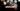# Leetcode | Solution of Defanging an IP Address

March 23rd, 2020
|

In this post, we will solve defanging an IP address from leetcode using two methods. Let's begin.

# Problem Statement

The question can be found at leetcode defanging an IP address problem.

The problem states that we need to replace all the occurrences of `.` in an IP address by `[.]`.

# Solutions

We'll solve this using two approaches

• Array Methods
• Regex

# Array Methods

The problem is very simple. In this approach, we'll split the IP string by `.`. It will convert the string in an array, which we'll join again by `[.]` and that would give us our desired output.

Let's see the code for this approach.

``````var defangIPaddr = function(address) {
};``````

Next, we'll solve the problem using regex.

# Regex

If we had a `replaceAll` method in JavaScript, we could have used that to solve the problem. However, we only get a `replace` method which replaces the first occurrence of the provided argument.

Thus, we'll use regex along with `replace` method, to replace all the occurrences of the `.` in the string.

Let's see the code for this approach.

``````var defangIPaddr = function(address) {
};``````

Here, we are using the replace method and are providing a global regex to search and replace in the argument.

`/.../` represents a regex expression, and `g` at the end means a global search. `\.` means we need to search for a `.`, but it is escaped because regex has a special meaning for `.`.

The given regex expression will search for all the occurrences of `.` and replace it with `[.]`.

# Summary

So, we solved the defanging an IP address problem by using array methods and regex expression.

I hope you enjoyed solving this question. This is it for this one, complete source code for this post can be found on my Github Repo. Will see you in the next one.